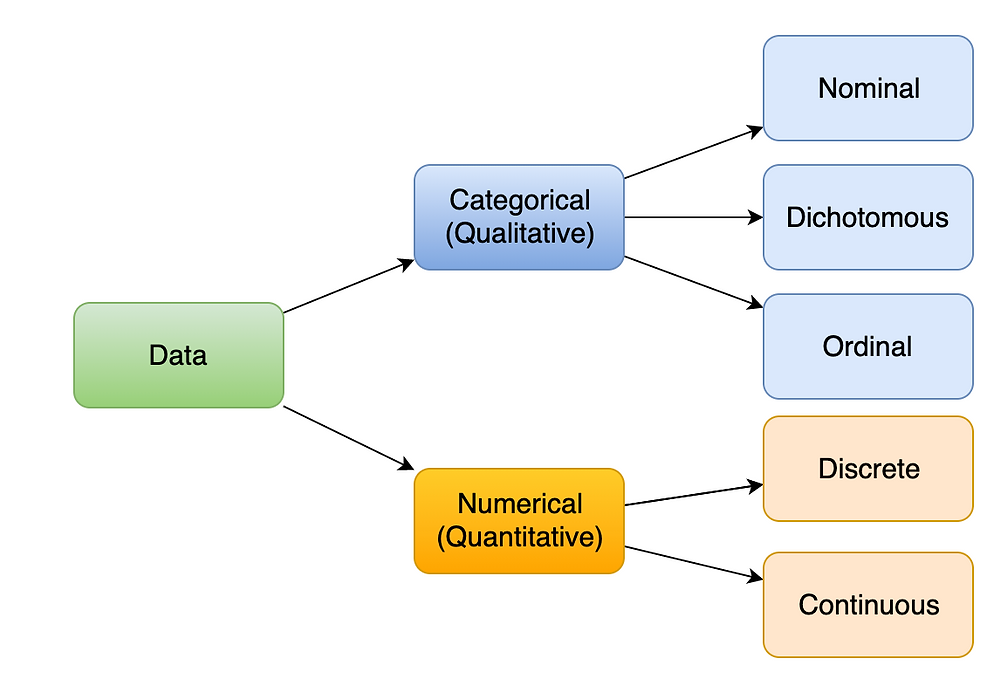Search
•Sai Geetha M N

# Types of Variables - Definition

There are different characteristics of data that are used for analysis and machine learning.

One very fundamental characteristic is whether the data is numeric or a category defined by discrete values. Based on this, there are two main types:

• Categorical data

• Numerical data

Categorical data is any data that has discrete levels or categories. For example, the country of a customer. It can have only specific values out of all the countries of the world. Another example could be gender.

Within the categorical variables, we have three different types:

1. Nominal

2. Dichotomous

3. Ordinal

Nominal comes from "name". So, the 'Country' example mentioned above is nominal data. It literally gives the names of countries and does not have any inherent order to be maintained in the data.

Dichotomous data is that which has only two values. Gender is an example of dichotomous data

Ordinal variables are those that have discrete values with an inherent 'order' in them. For example income groups such a low, medium, high, or education level which consists of high school, graduation, post-graduation, Ph.D.

Numeric data is data that speaks through numbers and is quantitative in nature.

Numeric data itself can be

1. Discrete

2. Continuous

Discrete data, as the term implies is numeric data that has only particular numbers allowed. Examples would be like the number of cars owned or the number of children. Usually, it is a set of discrete whole numbers.

Continuous data, on the other hand, are those that are numerically measured. It can have an infinite number of values. Typical examples for this are height, distance, age. They can have values like 1.235 meters or 43.234 years old etc.

Summarised in the figure below:The type of variable influences your analysis, data preparation, and the machine learning algorithms that you use. Very essential to understand the type of your target variable and the independent variables, in order to use the right techniques.